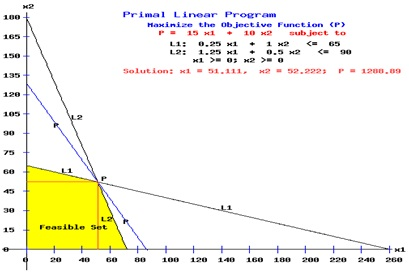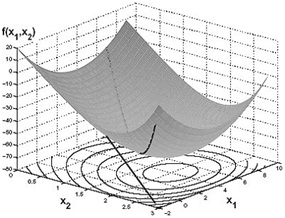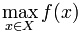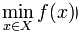#### Linear Programming Homework Help - K-12 Grade Level, College Level Mathematics

Introduction of Linear programming

The Linear programming or LP or linear optimization is a mathematical technique for determining a manner to achieve the finest outcome (such as maximum profit or lowest cost) in a given mathematical model for a few list of necessities represented as linear relationships. The Linear programming is a specific case of mathematical programming (i.e., mathematical optimization).

More officially, linear programming is a method for the optimization of a linear objective function, subject to linear inequality and the linear equality constraints. Its feasible area is a convex polyhedron which is a set defined as the intersection of finitely several half spaces, each of which is defined via a linear inequality. The objective function of it is a real-valued affine function defined on this polyhedron. The Linear programming algorithms find a point in the polyhedron where this function has the minimum (or maximum) value whenever such a point exists.

The problems which can be expressed in canonical form are known as linear programs.

The canonical form is:

Maximize cTy

Subject to Ay< d

And y > 0

Here, y represents the vector of variables, c and d are the vectors of (known) coefficients, A is a matrix of coefficients and T is the matrix transpose. Expression to be minimized or maximized is known as the objective function (cTy in this situation). The inequalities Ay ≤ b is the constraints that specify a convex polytope over which the objective function is to be optimized. In this situation the two vectors are comparable if they have similar dimensions. When every entry in the first is less-than or equal-to the corresponding entry in the second then we can say that the first vector is less-than or equal-to the second vector.Nonlinear programming

In mathematics, nonlinear programming or NLP is the process of solving a system of equalities and inequalities, collectively termed constraints, over a set of not known real variables, along with an objective function to be maximized or minimized, where some of the constraints or the objective functions are nonlinear.Non-linear optimization problem (NLO)

The problem can be stated simply as:To maximize some variable like product throughput orto minimize a cost function where

f: Rn →R

X € Rn

s.t. (subject to)

hi (x) = 0, I € I = 1,...,p

gj (x) <= 0, j € J = 1,..., m

Email based Linear and Non linear Programming Homework Help -Assignment Help

Tutors at the www.tutorsglobe.com are committed to provide the best quality Linear and Non linear Programming homework help - assignment help. They use their experience, as they have solved thousands of the Linear and Non linear Programming assignments, which may help you to solve your complex Linear and Non linear Programming homework. You can find solutions for all the topics come under the Linear and Non linear Programming. The dedicated tutors provide eminence work on your Math homework help and devoted to provide K-12 level math to college level math help before the deadline mentioned by the student. Linear and Non linear Programming homework help is available here for the students of school, college and university. Tutors Globe assure for the best quality compliance to your homework. Compromise with quality is not in our dictionary. If we feel that we are not able to provide the homework help as per the deadline or given instruction by the student, we refund the money of the student without any delay.

Qualified and Experienced Linear and Non linear Programming Tutors at www.tutorsglobe.com

Tutors at the www.tutorsglobe.com take pledge to provide full satisfaction and assurance in Linear and Non linear Programming homework help. Students are getting Math homework help services across the globe with 100% satisfaction. We value all our service-users. We provide email based Linear and Non linear Programming homework help - assignment help. You can join us to ask queries 24x7 with live, experienced and qualified math tutors specialized in Linear and Non linear Programming.